2016

### Akriti Lal's Blog

October 2016# Pareto Chart in Tableau 10.0

Posted by Akriti Lal Oct 25, 2016

The Pareto principle is the 80-20 rule. It basically states that, roughly 80% of results come from 20% of the causes. For example, 80% of profits come from 20% of the products.

In Tableau, you can apply a table calculation to Profit data to create a chart that shows the percentage of total Profit that come from the top sub category. You can also see which subcategory account for 80% of total profit.

We will follow the steps to create a Pareto Chart in Tableau on sample Super Store Data set. We all know that Sample Superstore is a mock up data for a sample super store, so it may or may not be the case that the Data does not follow the Pareto Principle.

Let’s do it for the Sample Superstore data set that comes up with Tableau 10 and we will use Tableau Desktop 10.0 to validate if the Data set that we are looking into follows the Pareto Principle or not. So the Question that we are seeking an answer here is “Does 80% of the Profit is Contributed by 20% of the Products Sub Category

Step 1: Drag Subcategory onto Columns and Profit on to rows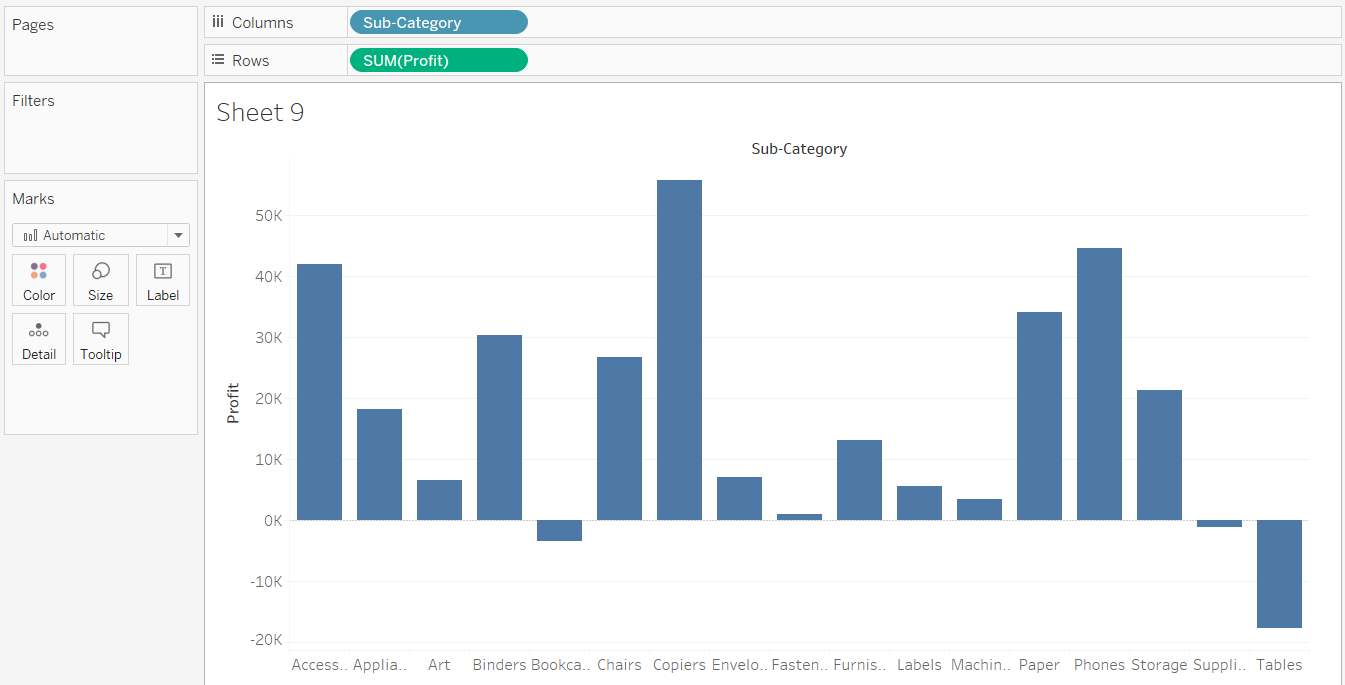Step 2: Sort in Descending Order on the basis of profit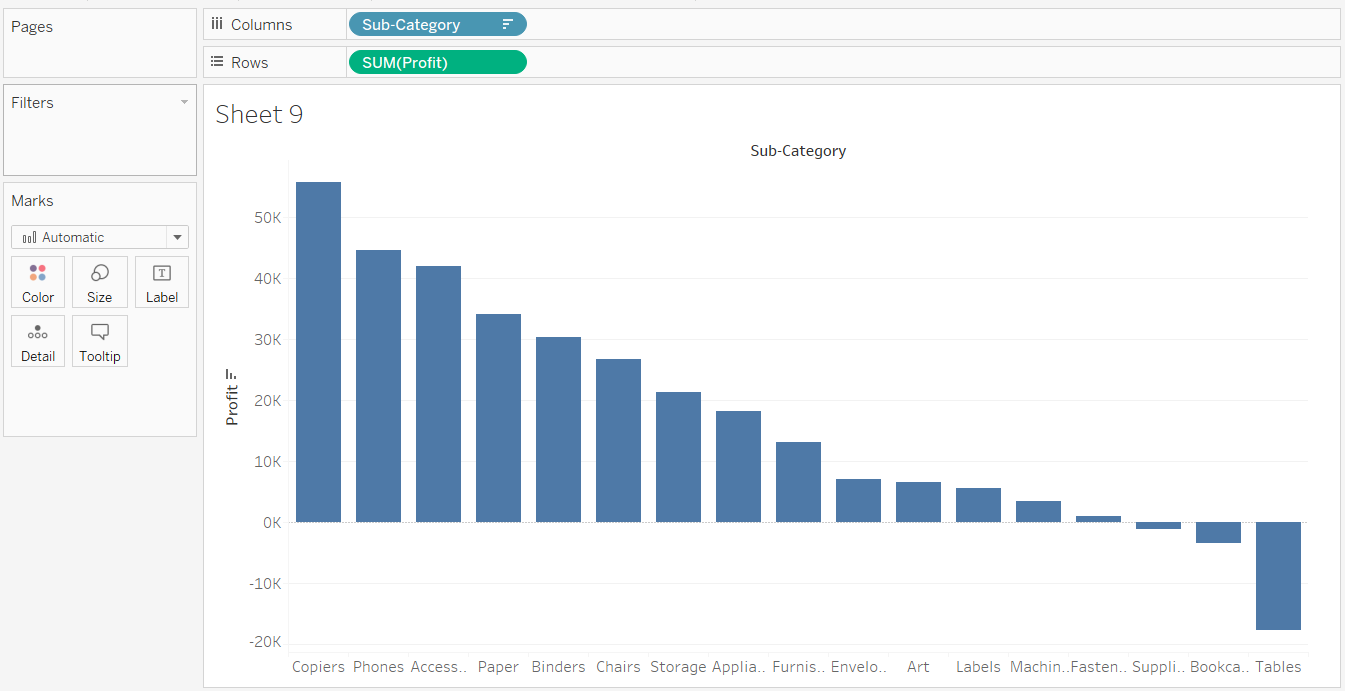Step 3: Add a Table Calculation (Running Total) of Sum of Profit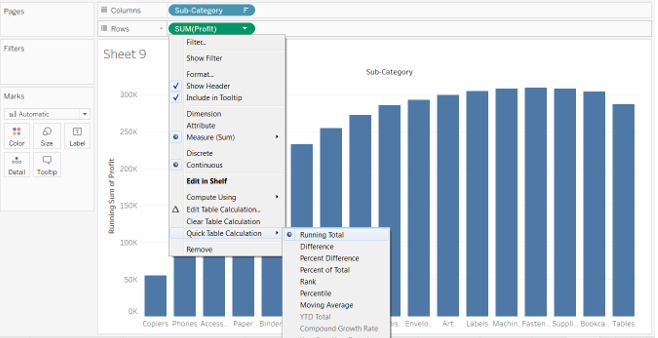So what we get till now is we have the “Running Total of Sum of Profit” i.e. Profit starts and the running total gives 100% of the profit that happened. So in order to know when 80% of the profit was done , we add a new secondary Table calculation on sum(profit) and change it to %.

Step 4: Change Running Total of Sum(Profit) from Actual Value to %. For this we add another Table calculation to it as below.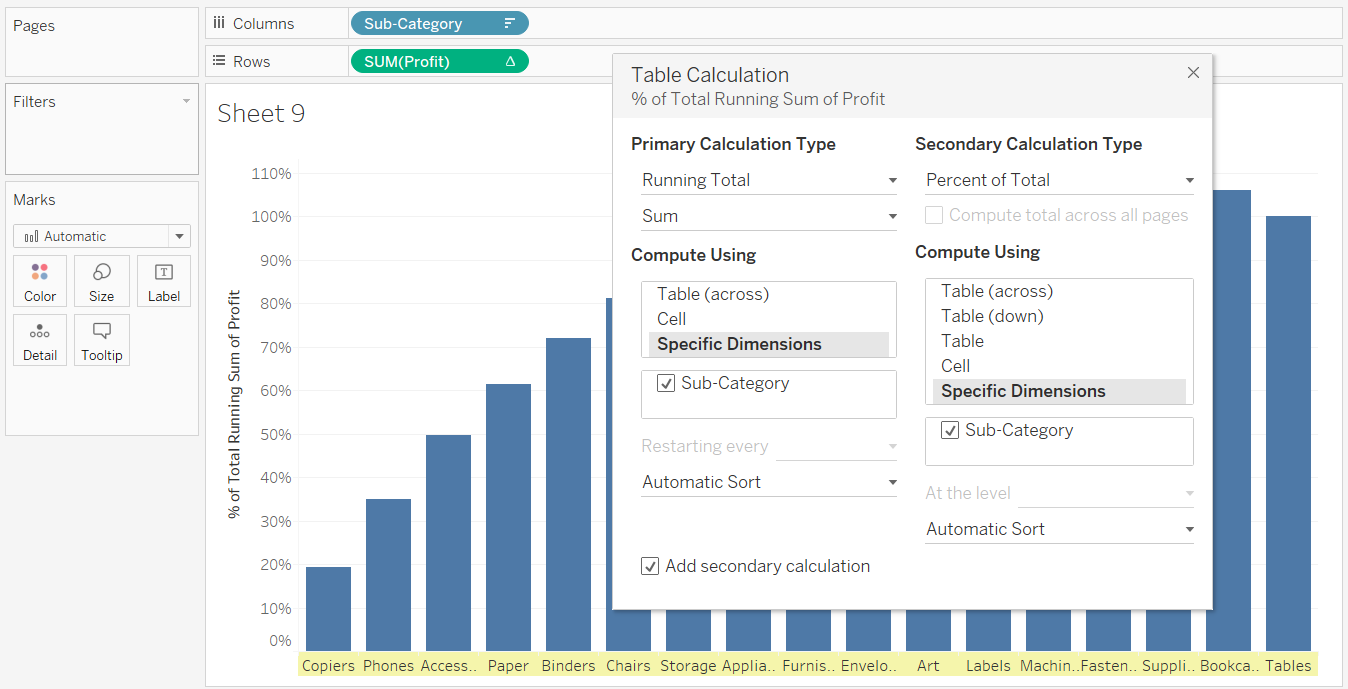Step 5: Analyze the Chart that you created. Pareto Chart is ready but the Analysis remains.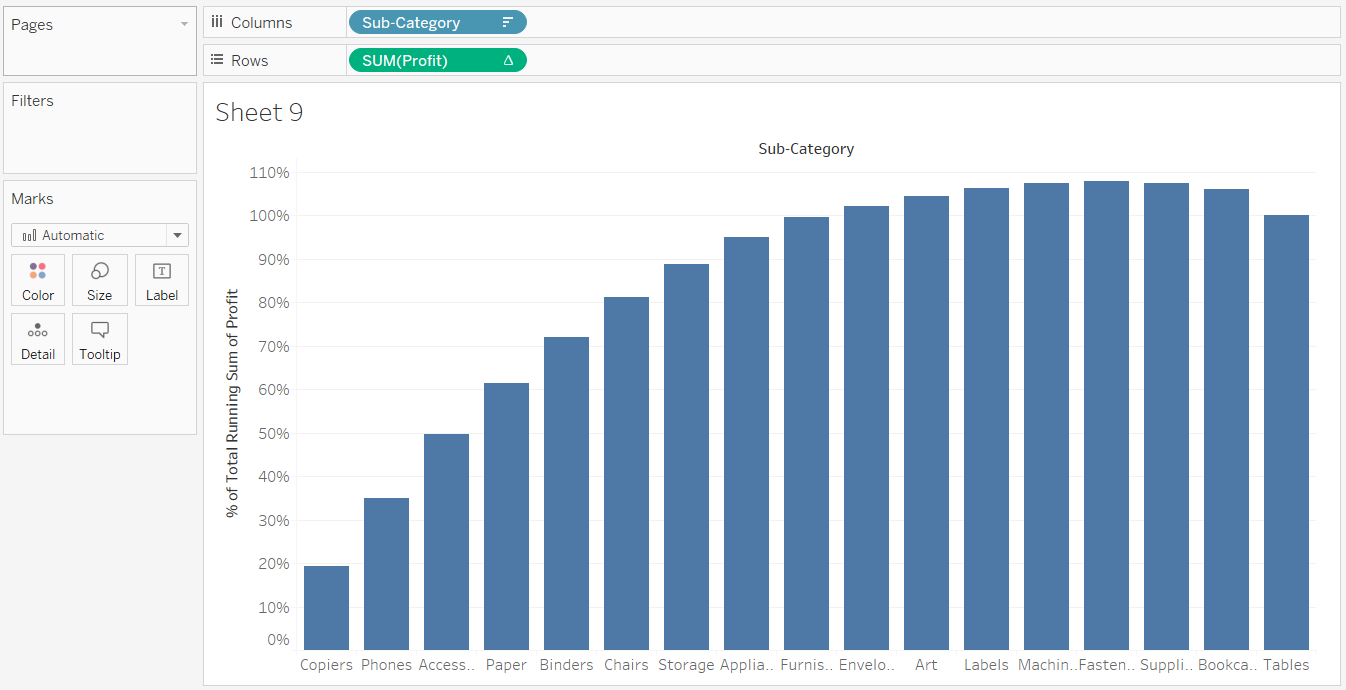So as we can see in the screen above, we can see the 80% of sales ( on the Y axis ) . And even without doing anything further we can see that 80% of sales on Y axis corresponds to 5 products of sub category i.e Copiers , Phones , Access, Paper , Binder, Chairs .

So out of total 17 Product Sub Category we have 6 product sub category that is reason for 80% of the profit and that makes 6/17 = 35% around. So we can say that Our Data does not follow the pareto principle as 80% of the Sales is being contributed by 35% of Product Sub Category and not 20% .

The Pareto Chart from Understanding perspective is complete, however if we want on the X- Axis instead of Product Sub Category Name we can show % so that, we can create trend lines of 80% on Y- Axis and 20% on X-axis to clearly see the 80-20 rule.

Now in order to calculate the % in x-axis , we have to do the same way as we calculate manually i.e. 6 / 17 i.e. index() / size () we will make a calculated field ParetoKPI and since we want to do it for “Sub Category” , we need to have the sub category in Marks shelf and compute Pareto KPI using “Sub Category”

Let’s do it in few steps as below

Step 6: To find out Percentage in x axis , create calculated field as ParetoKPI .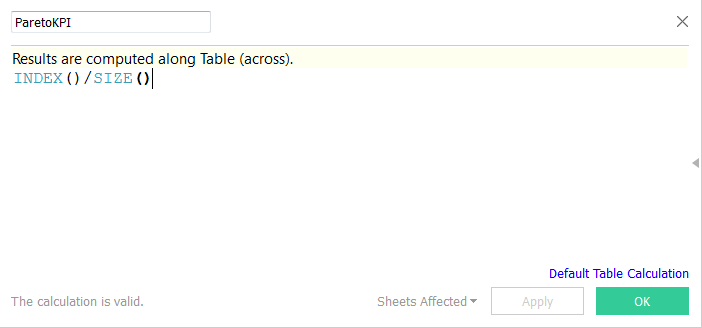Step 7: Thereafter Drag Sub Category from Columns to Mark Shelf and ParetoKPI to columns and for this Compute Using “Sub Category”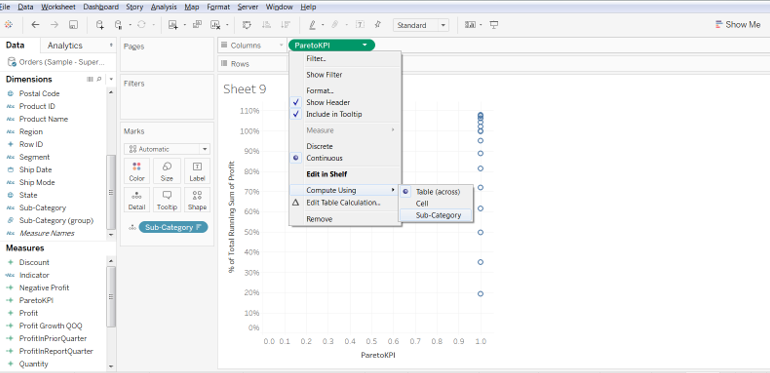Step 8:  In Marks Shelf change it to Line from Automatic and you get as below

So now we can see we have both Y axis and x axis in percent. We can format x axis to get in percentage.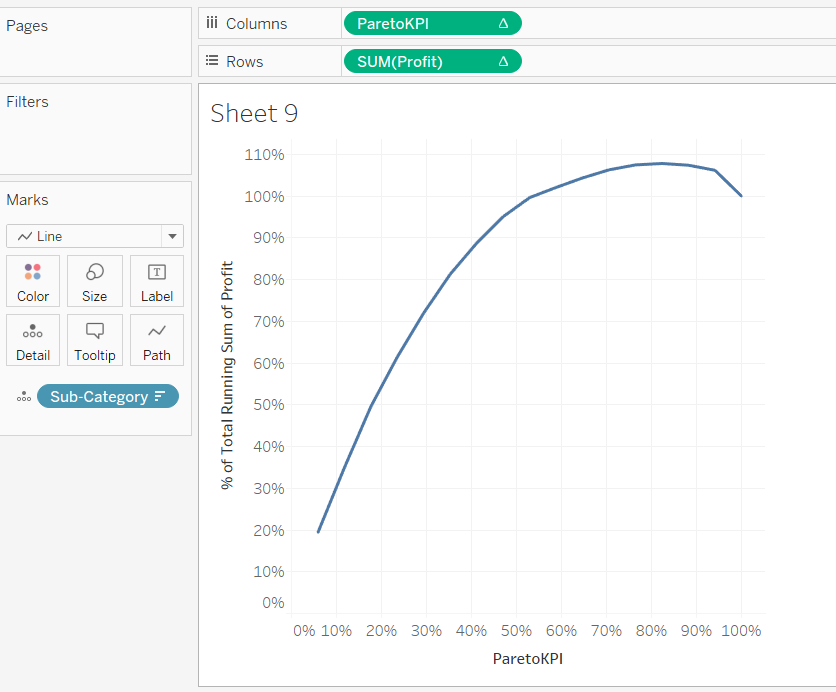Now we can drop 80% and 20% reference line on Yaxis and X axis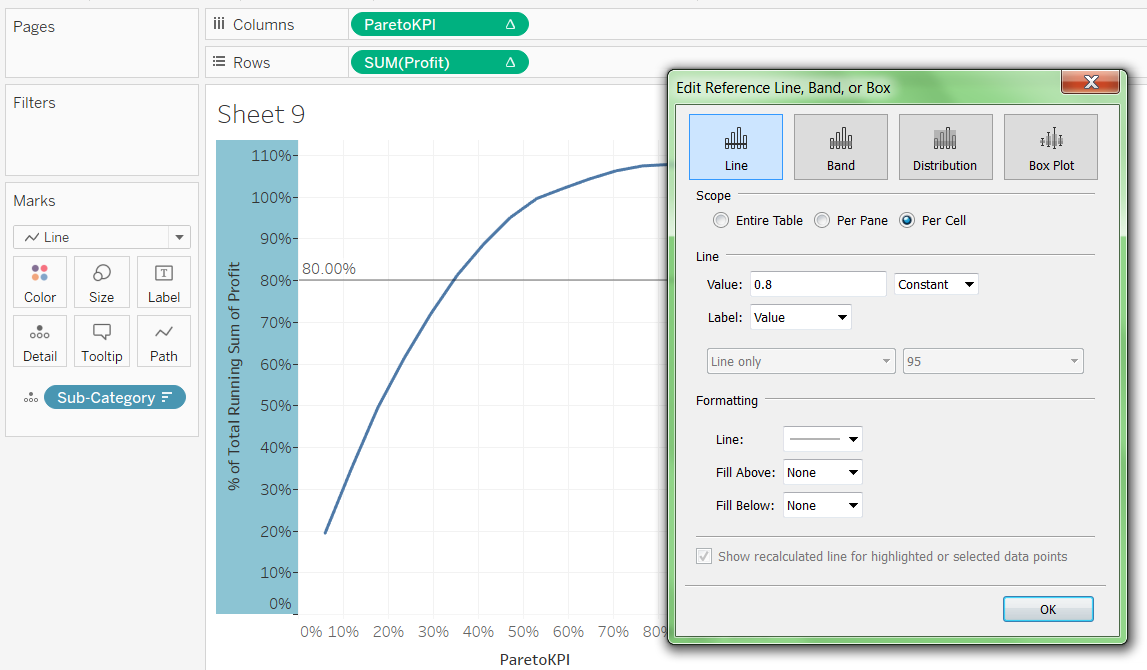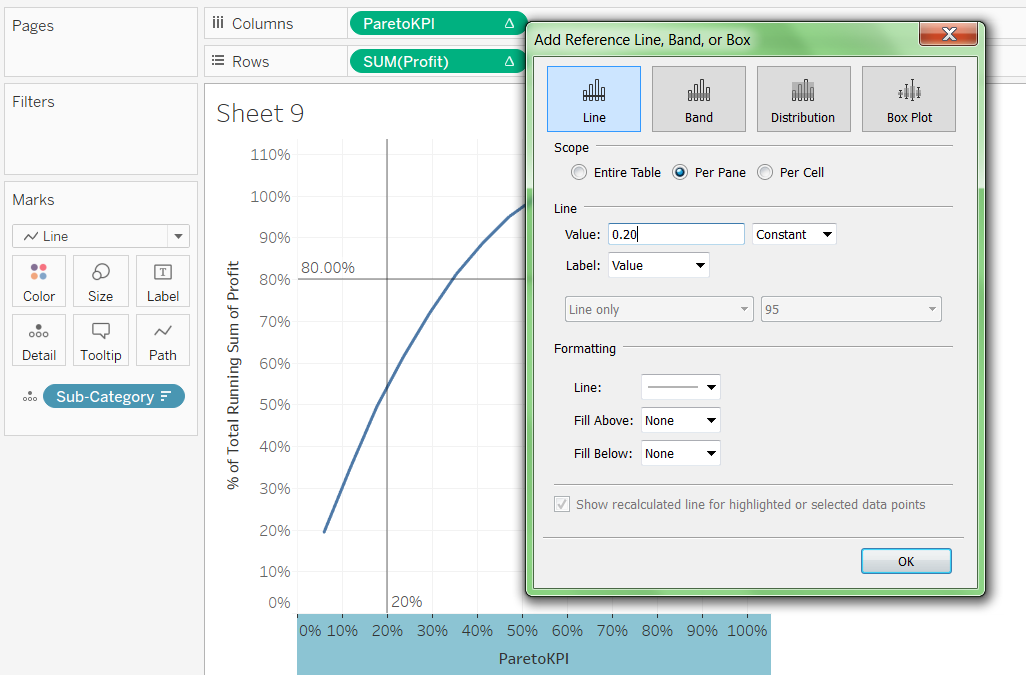So as we can see 20% of Product Sub Category leads to nearly 55% of the Profit. Since Sample Superstore is a mock up data , pareto principle does not satisfy. Even in real data ,whether Pareto principle satisfies or not depends on the How Data is distributed.# Using Pivot in Tableau 10.0

Posted by Akriti Lal Oct 4, 2016

A pivot table allows you to extract the significance from a large, detailed data set. You can pivot the data in your Microsoft Excel, text file, and Google Sheets data sources from crosstab format into columnar format to more easily perform analysis. Or, if you are not working with the types of data listed, you can use custom SQL as an alternative way to pivot your data in Tableau.

To explain pivot in tableau let’s take help of an example:

Suppose we have conducted a survey of the popularity/ preference of washing machines and the result is the excel below: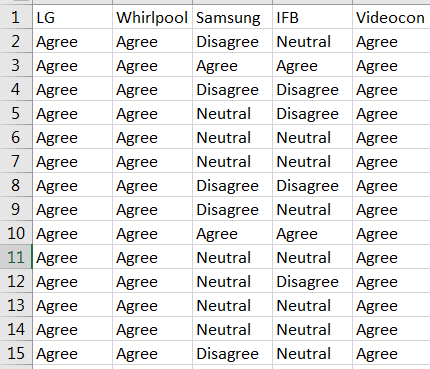So, now the requirement is that we want to analyze the feedback of each washing machine on the basis of Agree, Disagree and Neutral in Tableau.

Let’s connect Tableau to the excel and move ahead with the visualization: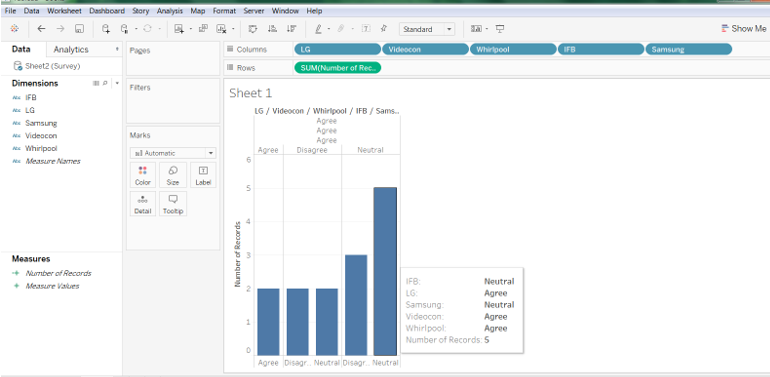The above visualization is not satisfying the requirement as the visualization is showing the total number of a particular combination ( i.e the highlighted Tableau visualization is showing that there are 5 records where we have a Neutral & an agree feedback). This is definitely not what we want.

In such scenarios the need of Pivot arises. To pivot in Tableau, follow along:

Step -1

After you have established you data source connection and have got the preview of the data; click on Manage Metadata pill to get the below visualization: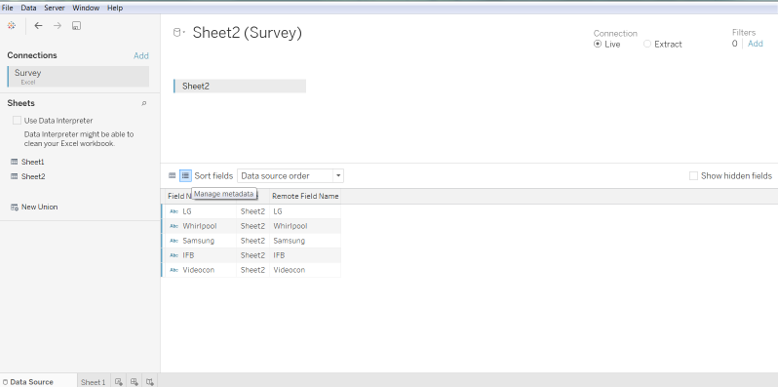Step-2

Pivot the table as below: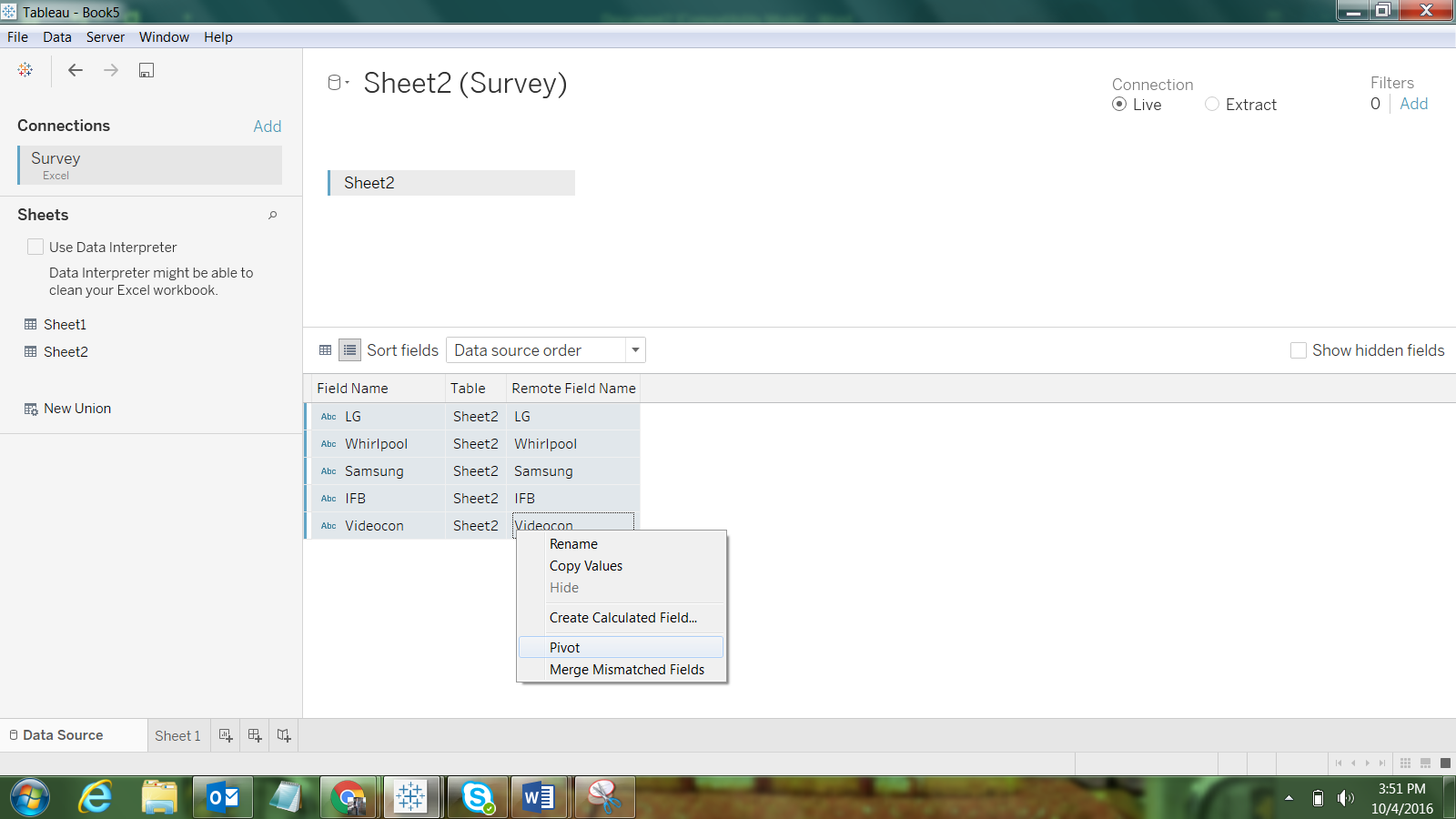Step-3:

The pivot result will be like this: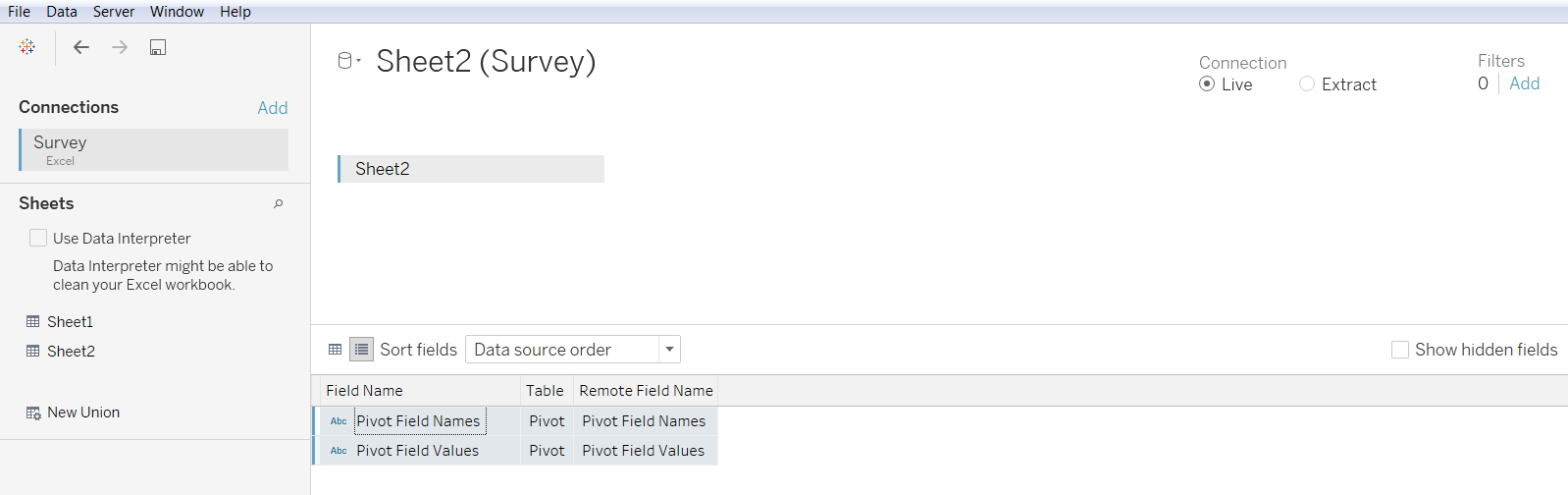The original fields in the data source are replaced with new fields called “Pivot field names” and “Pivot field values.” You can always rename the new pivot fields.

Step-4:

Finally, the Tableau Visualization which will state the individual feedback of the Washing Machines: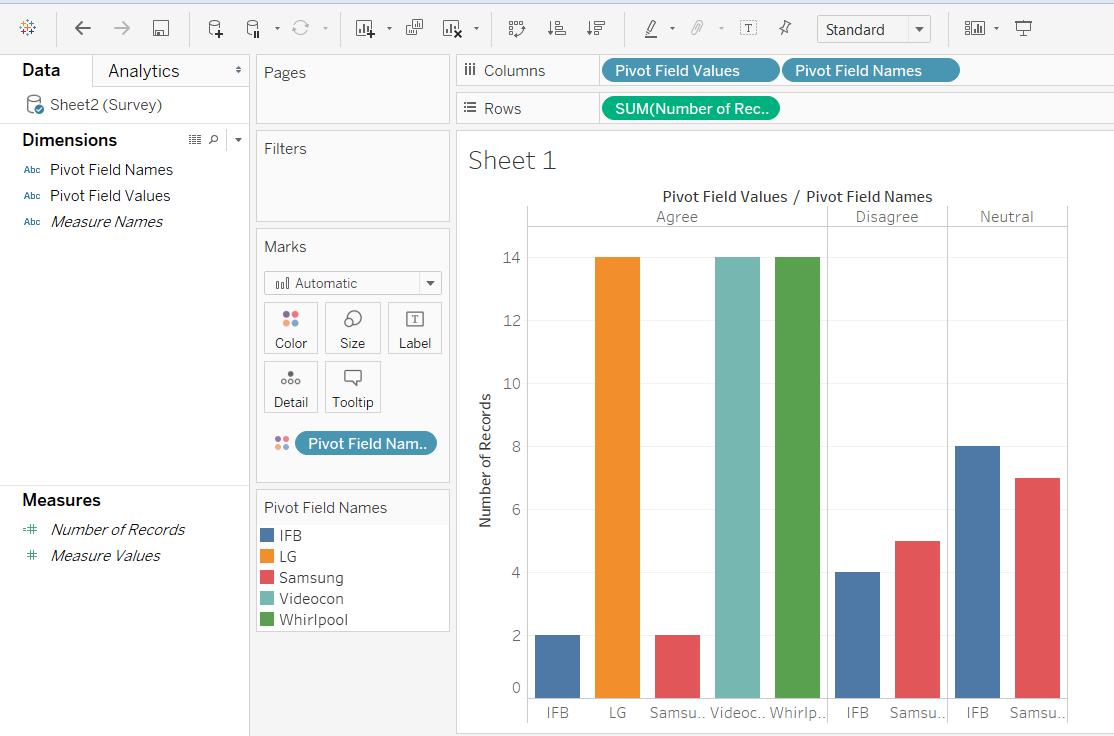Few points to remember while working with Pivot:

At a glance: Working with pivots

• The Pivot option is available from the grid and metadata grid.
• All fields in the pivot must be from the same connection.
• Only one pivot is allowed per data source.
• Pivot fields can be used as the join key.
• The Pivot option cannot be used in calculated fields.

Troubleshooting pivots

• Red fields in the view and fields with exclamation points in the Data pane: Because the original fields are replaced with new pivot fields, any references to the original fields in the view will no longer work. They cause fields to become red in the view or show a red exclamation point next to the field in the Data pane.
• Null values in the grid: If all of the original fields used in the pivot are removed, for example in an extract refresh, null values display in the pivot fields.# Waterfall Chart in Tableau 10.0

Posted by Akriti Lal Oct 3, 2016

Waterfall Chart in Tableau 10.0

Waterfall charts effectively display the cumulative effect of sequential positive and negative values. If you’re interested in showing where a value starts, ends and how it gets there incrementally, a waterfall chart is the best for you. You can also call a waterfall chart to be one of the best ways to unpack a large number and to break it down into digestible bites - maybe by organizational unit (very common) or by time period (also common, but less typically for a waterfall chart).

So, now that you have understood what a Waterfall Chart is let’s have a quick Hands-On.

Let’s make a visualization with Discrete MONTH (Order Date) and Sum[Profit], using Sample Superstore- xls. dataset, as we are well acquainted with this and we do not waste much time in Data Understanding.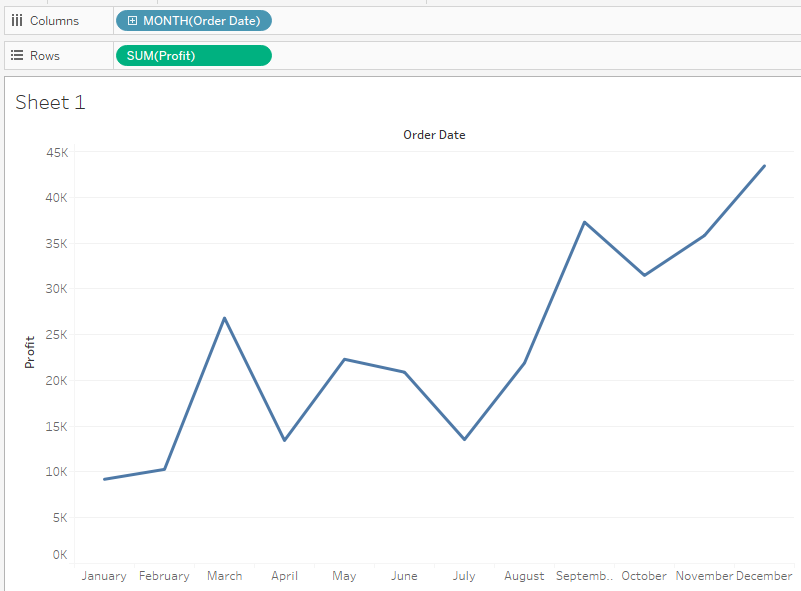Now, perform a Quick Table Calculation of Running Total on Profit.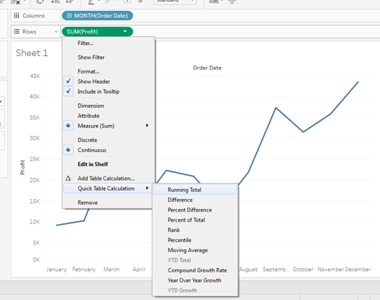Your visualization will change like below. Also note the change in Sum[Profit] in the Row Shelf stating it has a Table calculation performed to it, as it appears with a delta mark now.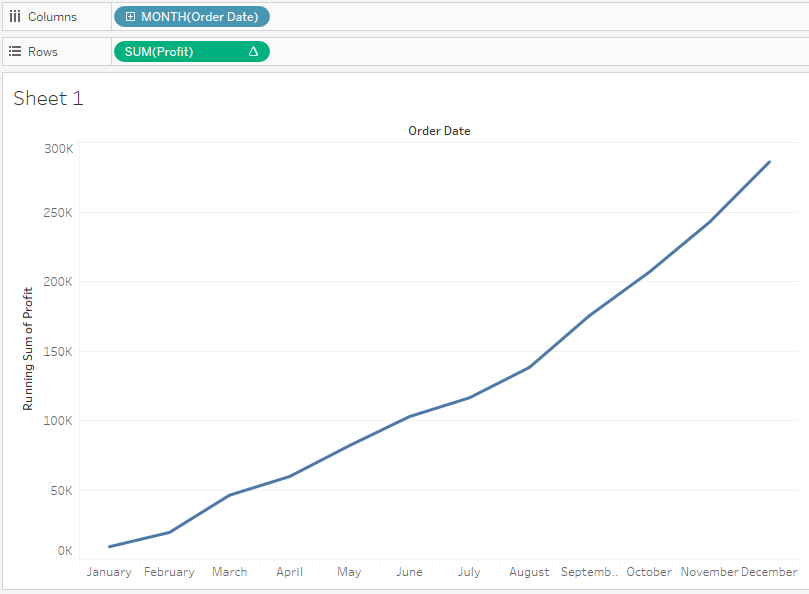From the Marks Shelf change the marks to Gant Chart. The change will result in below visualization: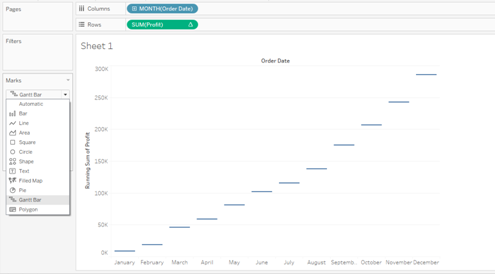This draws a line at the value of each Month’s Running Total. Now, we need to stretch each bar by Negative Profit i.e the Negative line will stretch upward to match the starting to the Positive Band and if the band is Positive then it has to stretch downwards to match the starting of the Negative band. To achieve this lets make a Calculated Field and call it Negative Profit.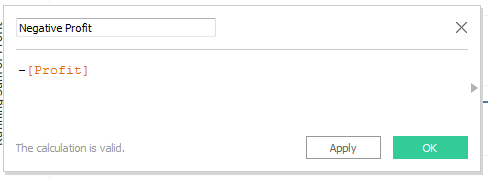Drag the new created field to the Size Marks Shelf and your resulting visualization will be as below: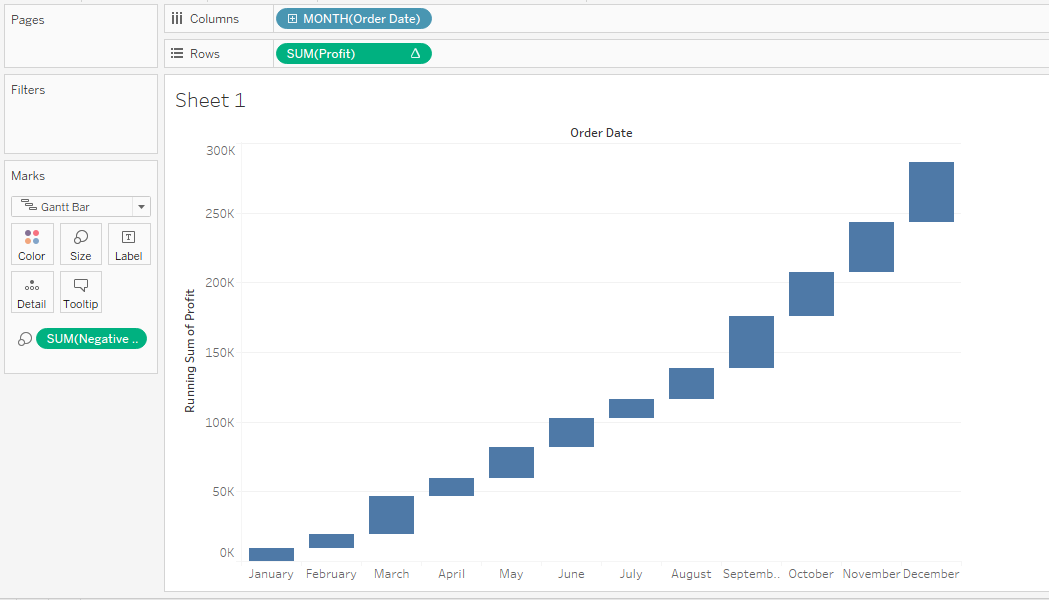Next Move the Profit from the Data pane to the Color Shelf and give it Stepped Size of 2 and change the color to Red-Green.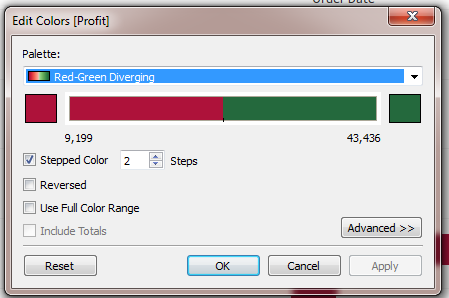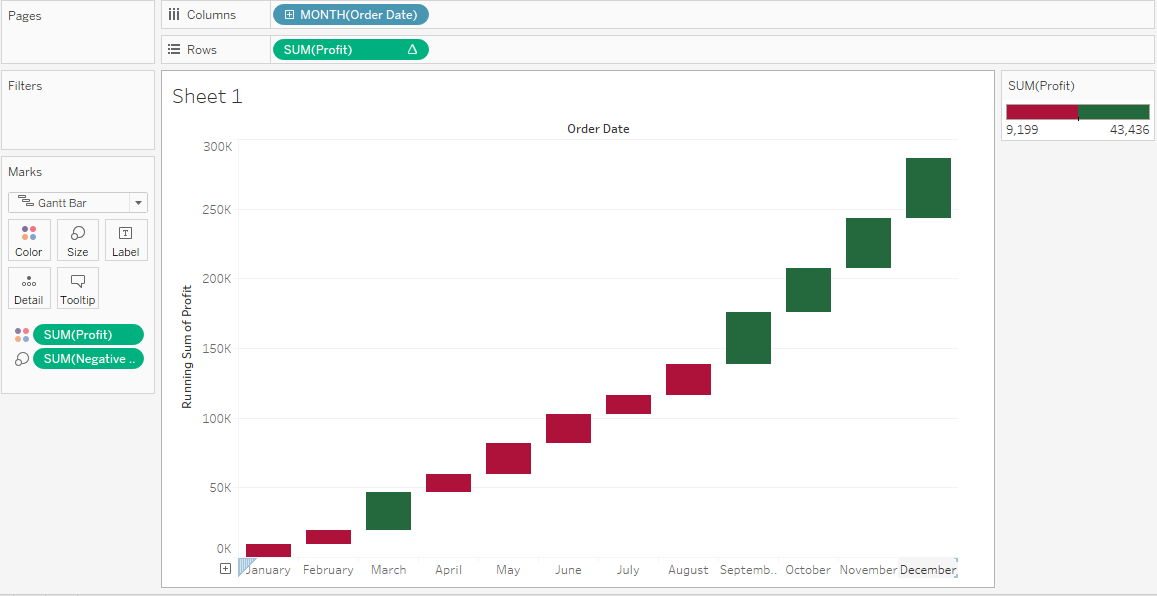By date: By tag: